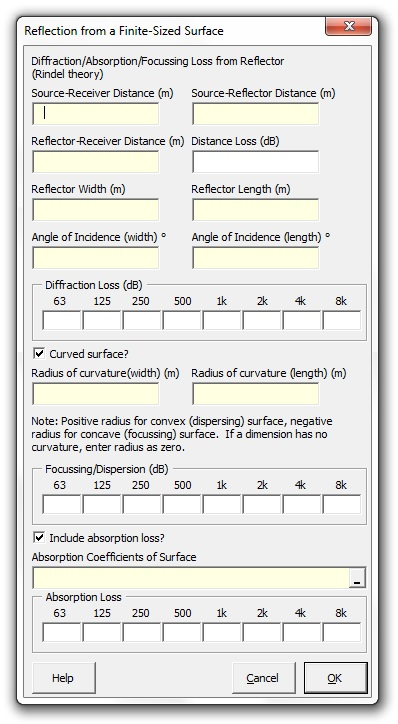### Strutt Help

Reflection from a Finite-Sized Surface    1/1, 1/3

Strutt|Auditorium Acoustics|Reflection from Finite Surface calculates the reflection strength from a finite-sized reflecting surface taking into account the reflector geometry and surface finish.The overall strength of the reflected level (relative to the direct sound) is calculated as follows:

Delta L_("total")=Delta L_("distance") + Delta L_("diffraction") + Delta L_("absorption") + Delta L_("curvature")

The distance loss is calculated as:

Delta L_("distance") = -20log((d_1+d_2)/d)
where:
d_1 is the distance from the source to the reflector.
d_2 is the distance from the reflector to the receiver.
d is the distance from the source to the receiver.

The diffraction loss is based on the Rindel methods as implemented in Odeon and is calculated based on the major and minor dimensions a and b of the reflector.

The overall diffraction loss is given by:

DeltaL_("diffraction")=10log(K_a*K_b)

K_a and K_b are factors related to the cut-off frequencies f_a and f_b below which the reflector does not reflect efficiently.

K_a = {(1 \ " for " \ f>f_a
\ ),(f/f_a \ " for " f<=f_a \ ):}

K_b = {(1 \ " for " \ f>f_b
\ ),(f/f_b \ " for " f<=f_b \ ):}

This results in a diffraction loss of 0 dB above both cut-off frequencies.
Between f_a and f_b the diffraction loss has a slope of 3 dB/octave.
Below both cut-off frequencies the diffraction loss has a slope of 6 dB/octave.

The cutoff frequencies f_a and f_b are calculated from the panel dimensions, angles of incidence and the source-reflector-receiver geometry as follows:

f_a = (c d"*")/(2(a cos(theta))^2

f_b = (c d"*")/(2(b cos(phi))^2

where:
a,b are the dimensions of the reflector.
theta is the angle of incidence relative to reflector dimension a
phi is the angle of incidence relative to reflector dimension b
d"*"=(d_1 d_2)/(d_1+d_2) is the characteristic distance for diffraction from the reflector
c is the wave speed of sound (343 m/s)

The (optional) absorption loss is calculated as follows:

DeltaL_"absorption"=10log(1-alpha)

where:
alpha is the absorption coefficient of the reflector at the given frequency.

In cases where alpha>=1, the maximum value is set to alpha=0.99

Note that this term can also include the effect of scattering (by the surface finish of the reflector) by inputting an effective absorption coefficent:
alpha_"eff"=alpha+s, where s is the scattering coefficient of the reflector at the given frequency.

The (optional) change in level due to curvature is calculated as follows:

DeltaL_"curvature" = -10log(1+(d"*")/(R_a cos(theta)))-10log(1+(d"*")/(R_b cos(phi)))

where:
R_a//R_b is the curvature of the reflector surface in the a,b directions

Note that focussing (concave) surfaces have a negative sign of R_a,R_b; flat surfaces have R_a,R_b=0; dispersive (convex) surfaces have a positive sign of R_a,R_b

References:
Odeon technical manual, p6-76
AAc Tech Note: Attenuation of Sound Reflections from Plane and Curved Surfaces (After Rindel)